# Catch up on the latest AI articles# Improving The Accuracy Of Repeated Patterns By Convolutional Shaplet Transform

3 main points
✔️ Shapelets were found to be highly explanatory, but inferior in modeling accuracy
✔️ Kernel approaches, such as convolution, were found to be more accurate, but less explanatory
✔️ Combine dilation with shapelets to satisfy both

written by Antoine GuillaumeChristel VrainElloumi Wael
(Submitted on 28 Sep 2021)

Subjects:  Computer Vision and Pattern Recognition (cs.CV); Machine Learning (cs.LG)code：The images used in this article are from the paper, the introductory slides, or were created based on them.

## first of all

For example, it is common to see cases where a series of characteristic movements are repeated, such as driving a robot arm. There is a concept of shaplet that can treat a series of motions as a unit, but there seems to be room for improvement in the accuracy of modeling. In this paper, we make a proposal for such a domain.

Time series data are used in a variety of fields, and in order to use algorithms safely in the field, it is sometimes equally important to solve problems efficiently as it is to understand the reasons behind decisions. Recent research in time series classification has produced algorithms with high accuracy at the expense of poor time efficiency. Convolutional kernel approaches, which have similar performance but extremely high scalability, are a step in that direction. However, none of these methods, especially the kernel approaches, are easy to interpret. On the other hand, methods based on shapelets, while not as good as these approaches, can produce results that are easy to understand for domain experts.

In this paper, we introduce the Convolutional Shapelet Transform (CST), which is an adaptation of time-series shaplets, introducing the concept of dilation (used in convolutional kernels) to shaplets. We also propose an algorithm to extract discriminative convolutional shaplets from convolutional kernels. This algorithm allows us to generate a small non-contiguous discriminative subsequence, independent of the length of the time series. We show that this approach is a novel shaplet-based algorithm that not only competes with state-of-the-art shaplet methods, but can also be viewed as a tool for interpreting convolutional kernel methods. The contributions of this paper can be summarized as follows.

- Enabled the use of extended time series shapelets.

- A method for extracting convolutional shaplets that relies on the output of convolutional kernels and their data identification capabilities.

- A comparative study with the most important shaplet and convolutional kernel methods.

- Method for providing interpretability of convolutional kernels

An example has been given to give an intuitive understanding of this paper. It is a visual example using the classic "GunPoint" dataset  (see Figure 1). This dataset is based on a sensor recording the movement of one hand of the actor. Class 0 represents the actor taking out the gun, pointing it, and putting it back in the holster, while class 1 represents the actor performing the same movements without taking out the gun.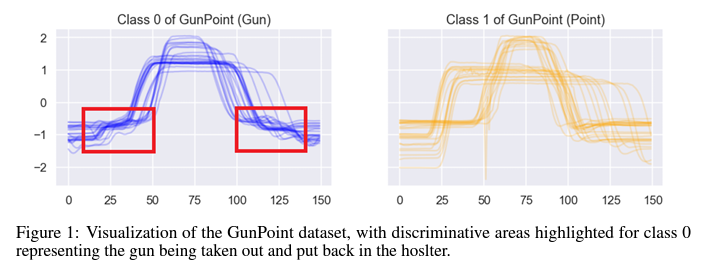## background

As background, we will focus on two methods that are at the core of the approach we present.

### shapette

A shaplet is defined as a time-series sub-sequence representing a class membership. In most cases, shaplet-based algorithms are distinguished by three steps: generation, filtering, and evaluation of candidate shaplets. In the original approach, the shaplets are used to construct a "shaplet tree". Candidates are extracted by enumerating all sub-sequences of length l that exist in the time series of the tree's nodes. The distance to the input is then computed and evaluated, and the best candidate for splitting the current node is selected by information gain: S = {v1,... ,vl} be a shaplet of length l, z-normalize the values (i.e., µS = 0 and σS = 1), and let X = {x1,... ,xn} be a time series, the shaplet distance is the minimum distance between S and all z-normalized sub-sequences of length l of X.

In the shaplet transform algorithm, we avoid evaluating the shaplets at each step of the tree, instead, we extract M shaplet candidates from N input time series and then evaluate them only once, resulting in an N × M distance matrix as input data for the classifier. This matrix is called the "shaplet transform".Figure 2 shows an example of the case with two shaplets. Many algorithms have been designed based on shaplets, including those that use discretization and random selection to filter out candidate shaplets, and those that generate a limited number of candidates by constructing a shaplet location index from the data. Some recent work includes shaplet locations as features for evolutionary approaches and neural networks, while others, like our method, extract shaplets from different representations of the input data.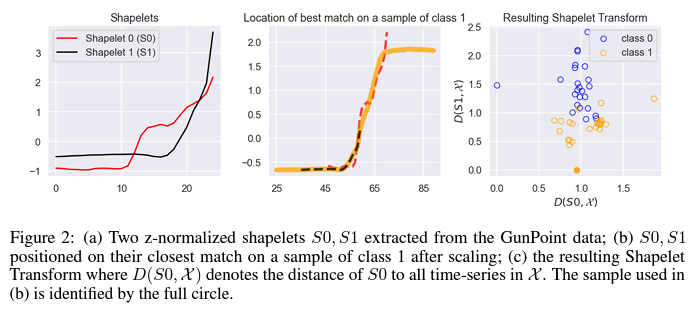### random convolution kernel transformation

The RandOm Convolutional KErnel Transfrom (ROCKET) randomly initializes a huge number of convolutional kernels (10.000 by default). Such kernels are K = {l, {w1,... , wl},b,d,p}, where l is the length of the kernel, w is the weight, b is the bias, d is the dilation (extension), and p is the padding. Using this kernel, features can be created from the convolution of the time series and fed to the classifier (Figure 3). Given a time series X ={x1,.... , xn} and a kernel K, the convolution produces a vector C of size n-((l-1)×d)+2p, where the position i of the vector is defined as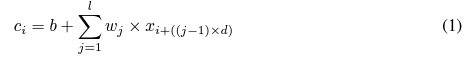In this context, a single point xi ∈ X can be used multiple times in computing C. (For example, xi can be used to compute any c ∈ (c0,... ,ci) can be used to compute C). If padding is used, add zeros to the beginning and end of x so that the kernels are centered at all points. Each kernel produces two features: the maximum value (max) and the fraction of positive values in the vector produced by the convolution (ppv). if N kernels are produced, the transformation outputs 2N features. mini-ROCKET is an almost deterministic and faster version of ROCKET. The weights are selected with {-1,2} and only ppv features are extracted.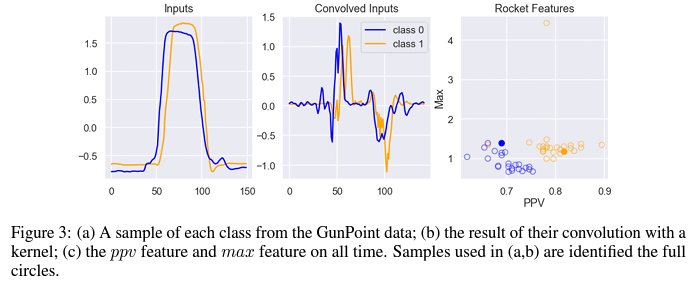In the following, given a sequence of doubles X and a kernel K, we denote by CK (X) the function that generates the convolution C, and call the convolution space for all X ∈ $\chi$ the set of CK (X).

## convolutional shapelet transformation

Given a kernel K, if the ppv statistic extracted from the convolutional space generated by K can partition the data into purer (in the sense of information gain) subsets, it is because it is the result of differences in the convolutional space resulting from patterns specific to each subset of the input space. Furthermore, since ppv is based on the proportion of points that are positive in the convolution, given the convolution space generated by K, if a point at index i makes a positive contribution to ppv in one subset of the data and a negative contribution in another subset, then the convolution used to compute this i-th value of It means that the points in the input space form a pattern that helps to identify the subsets. In this paper, we exploit the differences found in the convolution space to extract such discriminative patterns from the input space and construct a convolutional shaplet that compares time series based on the extracted patterns. We define the convolutional shaplet as S = {d,l, {v1,... , vl}}, where d is an augmentation parameter, l is the length, {v1,... ,vl} is the Z-normalized value. The shaplet distance of a time series X is defined by considering all subsequences of size l of X with an extension d between elements. The distance function D between the convolutional shaplet S and the time series X is written as follows:.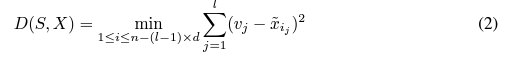Using this set of distance functions and convolutional shaplets, we can construct shaplet transforms that can be used in classification algorithms. Algorithm 1 assumes that X ={X1,.... ,Xn} as a set of input time series of even length, Y ={y1,... , yn} as the respective classes and outlines the approach.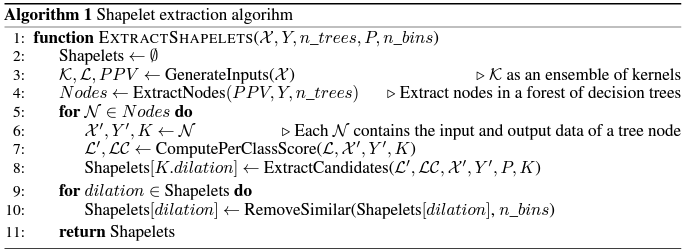### Kernel and data division generation

The first step is to generate a set of kernels from which we extract the ppv statistic and a matrix L that counts how many times each point of X appears in the convolution operation of CK (χ) that produces a positive value for each sample X ∈ χ and each kernel K ∈ Κ. The generation of the kernels relies on the same methodology as in Mini-ROCKET.

Once the input has been generated, the next step is to isolate the discriminative kernels that can be used to partition the data into purer subsets. To reflect this idea, the ExtractNodes function generates a forest of n_trees decision trees, featuring the ppv statistics of all kernels. It then extracts each node in the forest and stores the input data for this node, the two partitions that the node outputs, and the features (i.e., kernels) that were used to partition the node's input data. This allows us to explore the ensemble of nodes whose kernels are used to create a purer subset of the input data. Note that by using an ensemble of decision trees, we also remove duplicate nodes to account for potential draws.

### shapelet extraction

From the previous step, we obtain a matrix L and a set of tree nodes. Each node is represented by a kernel Ki that generated the ppv features used to partition the data, X the input time series used at that node, and Y the partitioning done at that node. Given the kernel Ki of a tree node, we identify the points in the convolutional space that produce a difference in ppv and extract the shaplet where this difference is the largest between the classes of Y. To compute this difference, we first need to find a score for each point in the convolutional space for each sample of X, and then construct a score for each class for which to compute the difference. This class score aims to measure the likelihood that a point in the convolution space leads to a positive value for this class of samples; to compute the score for each sample of X, denoted by the matrix L, we sum the contribution of all the points used in each convolution operation in the convolution space of all the points used in each convolution operation in the convolution space, denoted by the matrix L, are summed up. This yields a matrix L of the form (|X|,|X| - (l - 1) × d), where L[j,c] is the score of the c-th point of the convolution of sample j with kernel Ki. From this matrix, the per-class score LC is obtained by summing L for each sample in a class. Given these two new matrices, in order to extract a candidate shapelet for each of the two classes in partition Y, the difference between the LC of a class and the LC of the other class is computed, resulting in a vector D for each class. As mentioned earlier, the intuition behind this operation is that the larger the value of a point in D, the more likely it is that at that point the Ki convolution will output a positive value for one class of samples and a negative value for the other class. We can then see where to extract the candidates by looking at the parts of D that are above percentile P, as shown in Figure 4. The extraction itself is done by targeting each region pre-selected at P, noting m for the index of the maximum value of the region, and searching for which sample j of the current class maximizes L[j,m]. If it is found, a candidate is created using the value of this sample used in Ki 's mth convolution operation.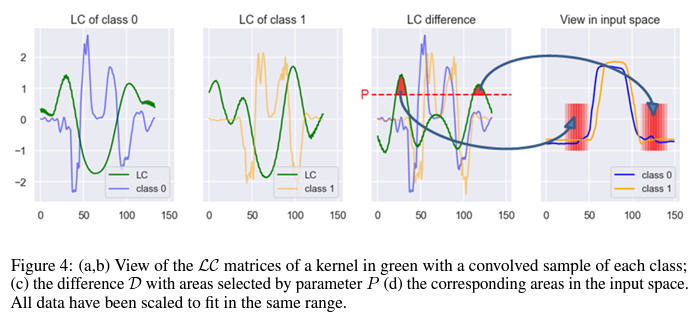Finally, the RemoveSimilar(Candidates,n_bins) function discretizes all candidate values that share the same expansion with uniformly spaced n_bins. Once all the candidate values are discretized, it removes the duplicate candidate values and keeps the other candidate values as a shaplet, which is used for the shaplet transform. We emphasize the following two points.

- The length of the convolutional shaplet does not depend on the length of the input time series, but only on the length of the kernel. Furthermore, the use of dilations removes the requirement that the shaplet be a continuous subsequence.

- Discretization reduces the number of candidates, produces shapelets that are not necessarily present in the input space, and limits overtraining.

## experiment

In the following, we use Ridge Classifier with Convolutional Shapelet Transform as the classifier and denote it as CST. We provide a python package using community standards to run our method on any dataset, as well as the scripts and data used in our experiments. We used 108 univariate data sets from the UCR archive for the comparative study, a subset of them for the sensitivity analysis, and two large data sets for the scalability analysis. Results display the average rank of objects using critical difference plots and add creeks (connections in horizontal bars) calculated using Wilcoxon-Holm post-hoc analysis. The cliques indicate that the differences between objects are not statistically significant. In the following, we compare CST with Shapelet Forest Classifier (SFC), MrSEQL, Shapelet Transform Classifier (STC) and Mini-ROCKET (MiniRKT).

### Sensitivity analysis of parameters

For bins, there were no significant differences among the comparisons made; for P, significant differences appear above 80. The number of trees is 250 anomalies, and no improvement is seen.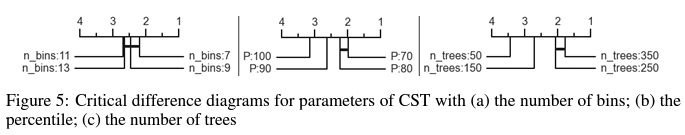### scalability

Figure 6 shows the average total execution time for each method, including shaplet extraction, distance computation, classification, and prediction, when the number of parallel threads is limited to 20 and 10 resamples are performed.The difference in execution time between SFC and CST is partly due to the fact that in the current version, tree node extraction and shaplet The difference is very small when compared with only one thread, partly because MrSEQL uses approximation techniques such as SAX in its algorithm, and is more efficient than CST for long time series, but this advantage does not apply to the number of samples.